# Magnetic Reluctance: What is it? (Formula, Units & Applications)

Contents

## What is Reluctance?

Magnetic reluctance (also known as reluctance, magnetic resistance, or a magnetic insulator) is defined as the opposition offered by a magnetic circuit to the production of magnetic flux. It is the property of the material that opposes the creation of magnetic flux in a magnetic circuit.

In an electrical circuit, the resistance opposes the flow of current in the circuit and it dissipates the electric energy. The magnetic reluctance in a magnetic circuit is analogous to the resistance in an electric circuit as it opposes the production of magnetic flux in a magnetic circuit but it does not give rise to the dissipation of energy rather it stores magnetic energy.

Reluctance is directly proportional to the length of the magnetic circuit and inversely proportional to the area of the cross-section of the magnetic path. It is a scalar quantity and denoted by S. Note that a scalar quantity is one which is fully described by a magnitude (or numerical value) only. No direction is required to define the scalar quantity.

Mathematically it can be expressed as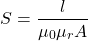where, l = length of the magnetic path in meters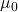= permeability of free space (vacuum) =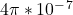Henry/meter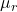= relative permeability of a magnetic material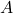= Cross sectional area in square meters (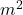)

In AC as well as DC magnetic fields, the reluctance is the ratio of the magnetomotive force (m.m.f) to the magnetic flux in a magnetic circuit. In a pulsating AC or DC field, the reluctance is also pulsating.

Thus it can be expressed as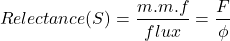### Reluctance in a Series Magnetic Circuit

Like in a series electrical circuit, the total resistance is equal to the sum of the individual resistances,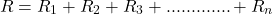Where,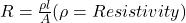Similarly, in a series of magnetic circuits, the total reluctance equals the sum of the individual reluctances encountered around the closed flux path.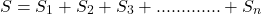Where,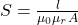### What is Permeability?

The permeability or magnetic permeability is defined as the ability of a material to allow the magnetic lines of force to pass through it. It helps the development of the magnetic field in a magnetic circuit.

The SI unit of permeability is Henry/meter (H/m).

Mathematically,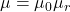H/m

Where,= permeability of free space (vacuum) =Henry/meter= relative permeability of a magnetic material

It is the ratio of magnetic flux density (B) to magnetizing force (H).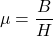### Relative Permeability

Relative Permeability is defined as the degree to which the material is a better conductor of magnetic flux as compared to free space.

It is denoted by.

## What is Reluctivity?

Reluctivity or a specific reluctance is defined as the reluctance offered by a magnetic circuit of a unit length and a unit cross-section.

We know the reluctance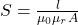When l = 1 m and A = 1 m2 then, we have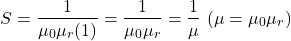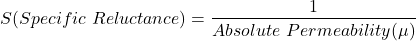Its unit is meter/Henry.

It is analogous to resistivity (specific resistance) in an electric circuit.

## Permeance vs Reluctance

Permeance is defined as the reciprocal of reluctance. It is denoted by P.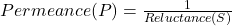## Reluctance Units

The unit of reluctance is ampere-turns per Weber (AT/Wb) or 1/Henry or H-1.

## Dimension of Magnetic Reluctance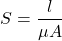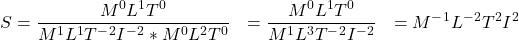## Reluctance Formula

(1)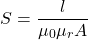Where,(In an electrical circuit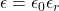)

Therefore,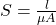Where,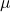= permeability of the magnetic material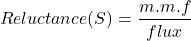(2)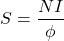Comparing Equation (1) and (2), we get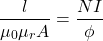Rearranging terms, we get

(3)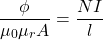But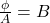and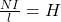put this into equation (3) we get,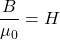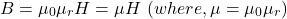### Magneto Motive Force (M.M.F)

M.M.F is defined as the force that tends to establish the flux through a magnetic circuit.

It is equal to the product of the current flowing through the coil and the number of turns of the coil.

Hence,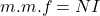Its unit is ampere-turns (AT).

Thus,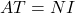The work done in carrying a unit magnetic pole (1 Wb) through the entire magnetic circuit is called a magnetomotive force (m.m.f).

It is analogous to electromotive force (e.m.f) in an electrical circuit.

## Applications of Reluctance

Some of the applications of reluctance include:

• In the transformer, reluctance is mainly used to reduce the effect of magnetic saturation. The constant air gaps in a transformer increase the reluctance of the circuit and hence store more magnetic energy before saturation.
• Reluctance motor is used for many constant speed applications such as electric clock timer, signaling devices, recording instruments, etc, which is works on the principle of variable reluctance.
• One of the main characteristics of the magnetically hard materials is that it has a strong magnetic reluctance which is used to create permanent magnets. Example: Tungsten steel, cobalt steel, chromium steel, alnico, etc….
• The speaker magnet is covered with a soft magnetic material such as soft iron to minimize the effect of the stray magnetic field.
• Multimedia loudspeakers are magnetically shielded in order to reduce magnetic interference caused to TV (televisions) and CRTs (Cathode Ray Tube).

Want To Learn Faster? 🎓
Get electrical articles delivered to your inbox every week.
No credit card required—it’s 100% free.